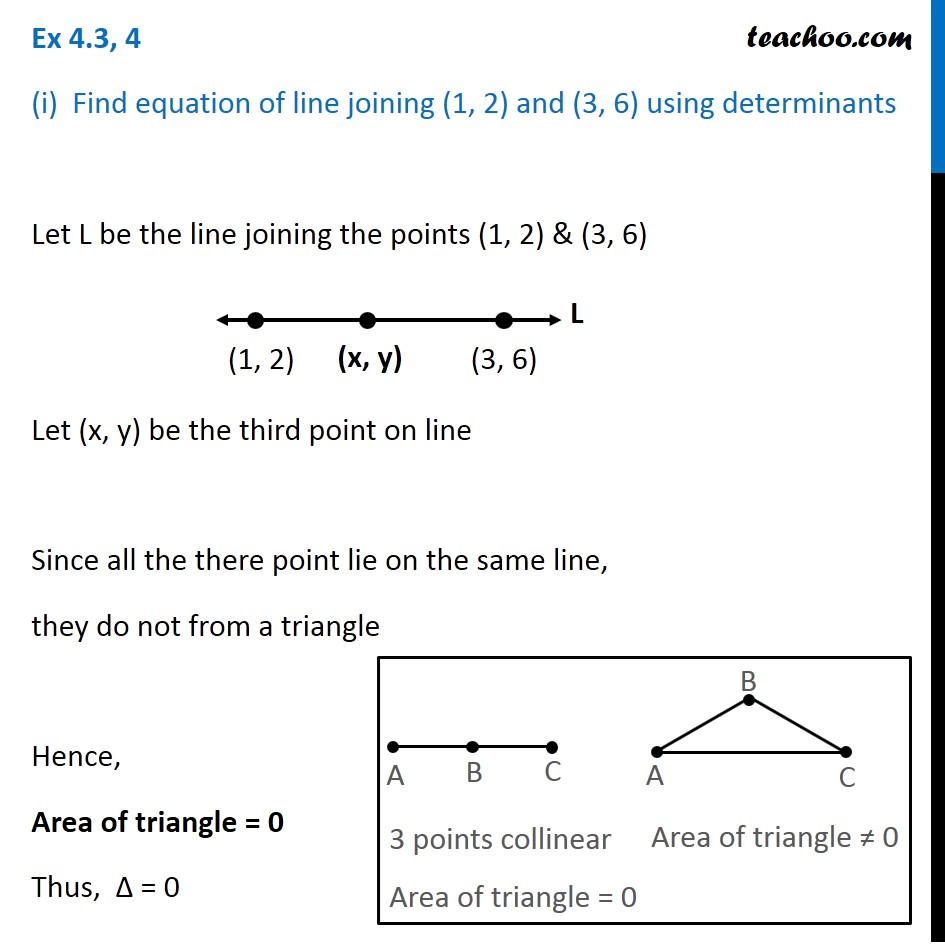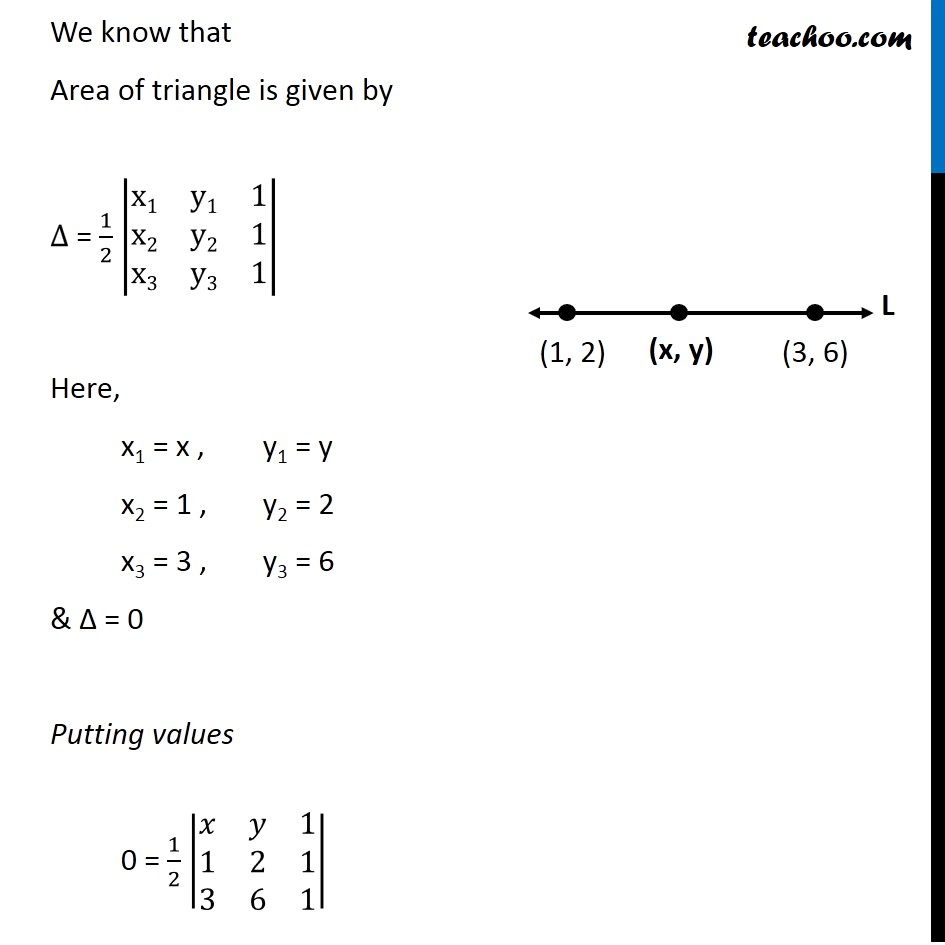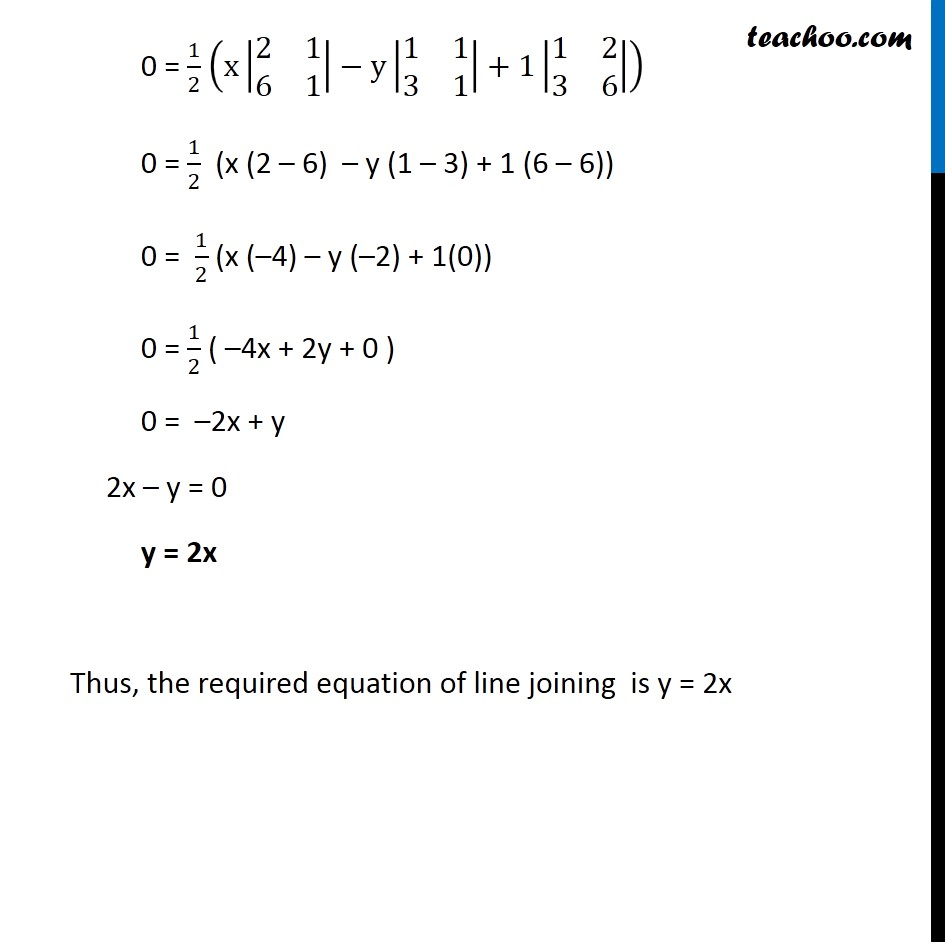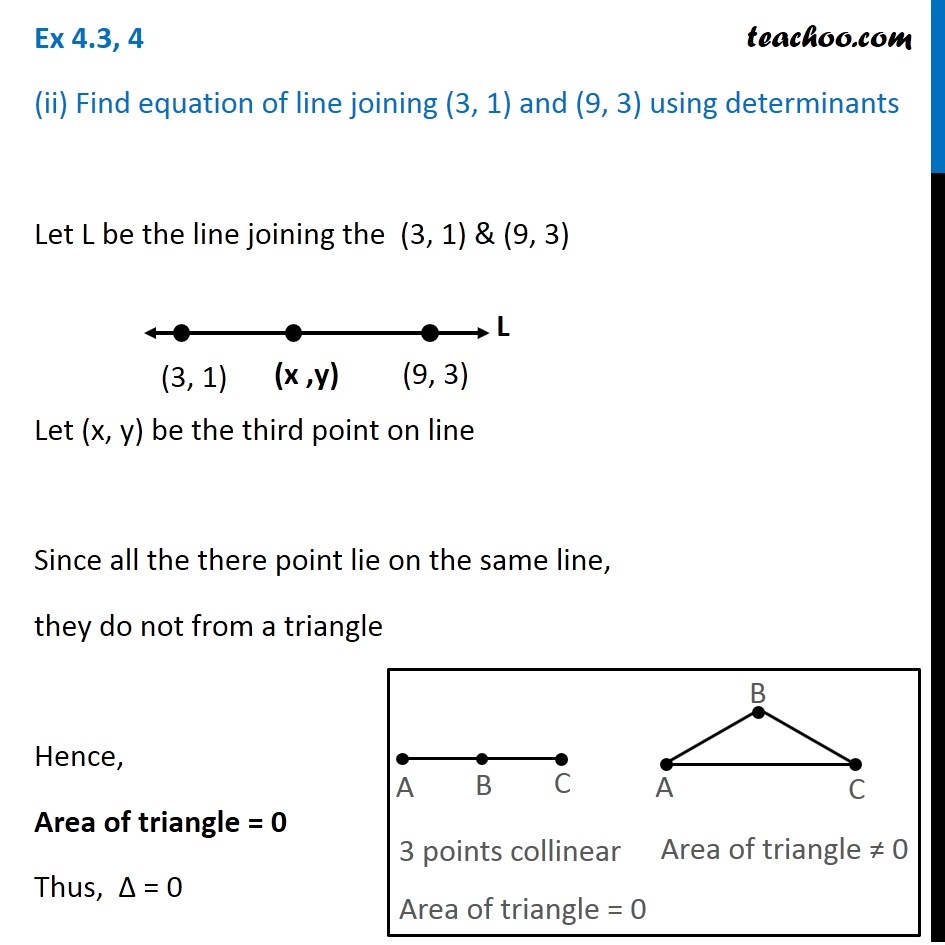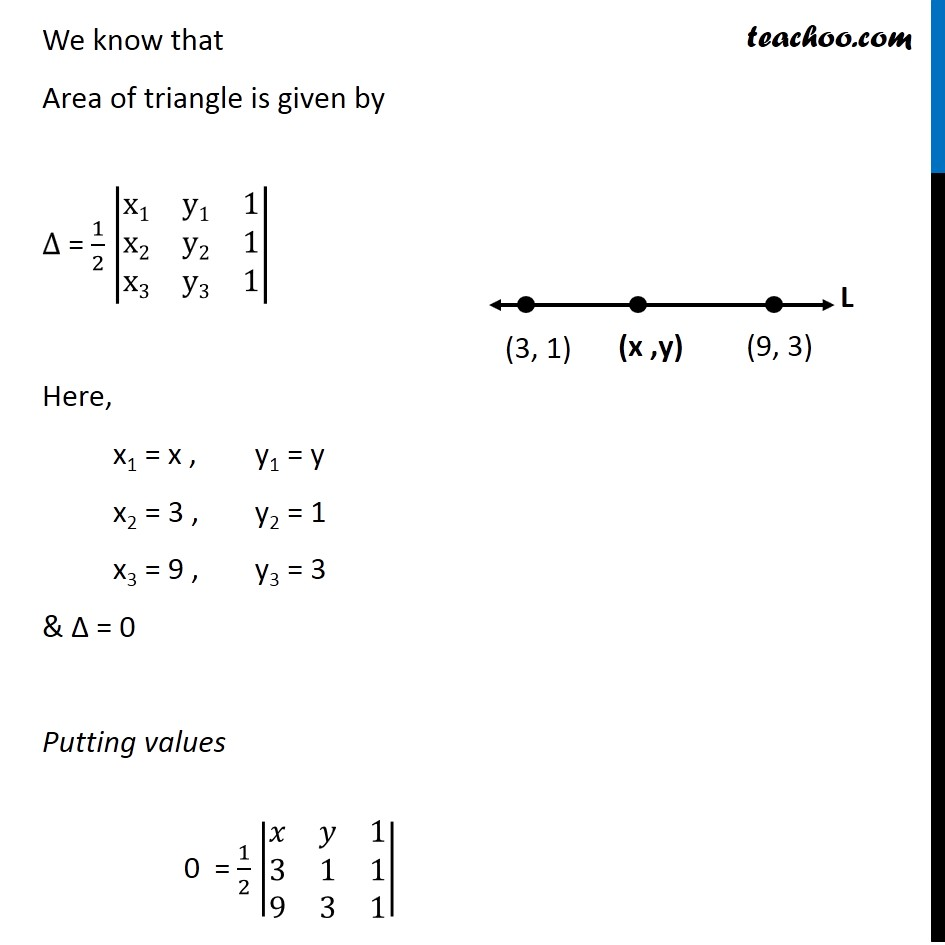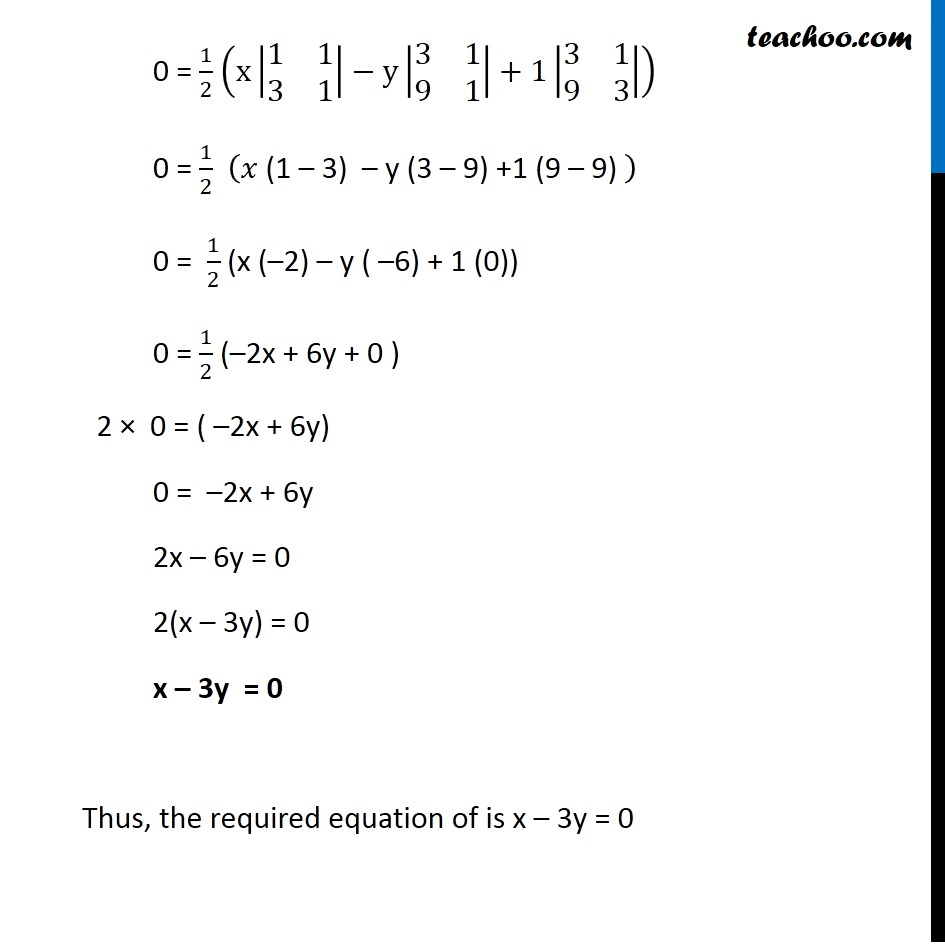Subscribe to our Youtube Channel - https://you.tube/teachoo

1. Chapter 4 Class 12 Determinants
2. Serial order wise
3. Ex 4.3

Transcript

Ex 4.3, 4 (i) Find equation of line joining (1, 2) and (3, 6) using determinants Let L be the line joining the points (1, 2) & (3, 6) Let (x, y) be the third point on line Since all the there point lie on the same line, they do not from a triangle Hence, Area of triangle = 0 Thus, ∆ = 0 3 points collinear Area of triangle = 0 Area of triangle ≠ 0 Area of triangle ≠ 0 We know that Area of triangle is given by ∆ = 1/2 |■8(x1&y1&1@x2&y2&1@x3&y3&1)| Here, x1 = x , y1 = y x2 = 1 , y2 = 2 x3 = 3 , y3 = 6 & ∆ = 0 Putting values 0 = 1/2 |■8(𝑥&𝑦&1@1&2&1@3&6&1)| 0 = 1/2 (x|■8(2&1@6&1)|−y|■8(1&1@3&1)|+1|■8(1&2@3&6)|) 0 = 1/2 (x (2 – 6) – y (1 – 3) + 1 (6 – 6)) 0 = 1/2 (x (–4) – y (–2) + 1(0)) 0 = 1/2 ( –4x + 2y + 0 ) 0 = –2x + y 2x – y = 0 y = 2x Thus, the required equation of line joining is y = 2x 3 points collinear Area of triangle = 0 Area of triangle ≠ 0 We know that Area of triangle is given by ∆ = 1/2 |■8(x1&y1&1@x2&y2&1@x3&y3&1)| Here, x1 = x , y1 = y x2 = 3 , y2 = 1 x3 = 9 , y3 = 3 & ∆ = 0 Putting values 0 = 1/2 |■8(𝑥&𝑦&1@3&1&1@9&3&1)| 0 = 1/2 (x|■8(1&1@3&1)|−y|■8(3&1@9&1)|+1|■8(3&1@9&3)|) 0 = 1/2 (𝑥 "(1 – 3) – y (3 – 9) +1 (9 – 9) " ) 0 = 1/2 (x (–2) – y ( –6) + 1 (0)) 0 = 1/2 (–2x + 6y + 0 ) 2 × 0 = ( –2x + 6y) 0 = –2x + 6y 2x – 6y = 0 2(x – 3y) = 0 x – 3y = 0 Thus, the required equation of is x – 3y = 0

Ex 4.3